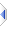# mod

 Definition: atom a3 = mod(atom a1, atom a2) Description: returns the remainder of two atoms using floored division. Comments: When the two operands have the same sign this returns the same result as remainder(). When the signs are different this function rounds away from zero whereas remainder rounds towards zero. The result has the same sign as a2, whereas the result of remainder() has the same sign as a1. This function may be applied to an atom or sq_mod() to all elements of a sequence. The rules for sequence operations apply. Note that the phix implementation of mod() tries to yield the same results as plain C, however if you look at the right-hand table (Integer modulo operators in various programming languages) on wikipedia you will see that the modulus operation is just about as far from a standard thing as anything could ever be. Example: ``` x,y: -9,-4 -9,+4 +9,-4 +9,+4 remainder(x,y): -1 -1 +1 +1 mod(x,y): -1 +3 -3 +1 -- (matches C's % operator) ``` Implementation: See builtins\pmaths.e (an autoinclude) for details of the actual implementation. See Also: remainder